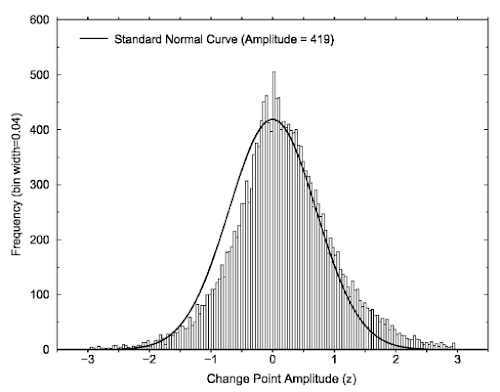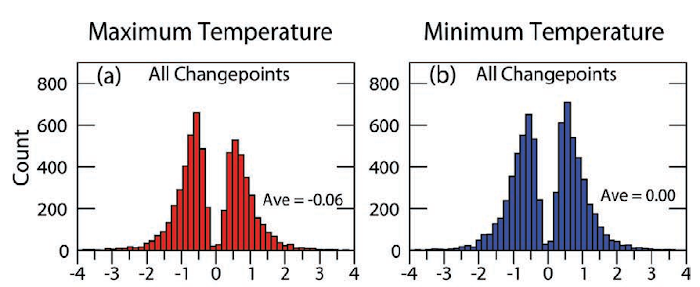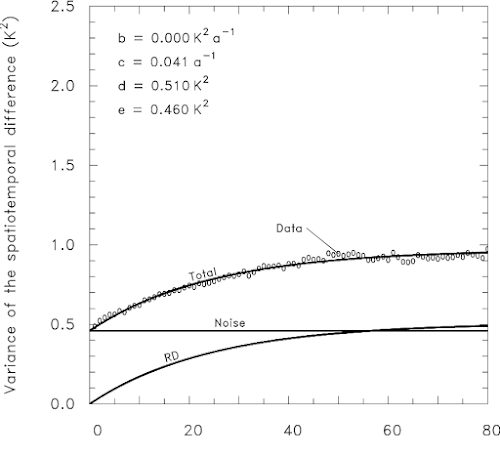## Monday, 24 February 2020

### Estimating the statistical properties of inhomogeneities without homogenization

One way to study inhomogeneities is to homogenize a dataset and study the corrections made. However, that way you only study the inhomogeneities that have been detected. Furthermore, it is always nice to have independent lines of evidence in an observational science. So in this recently published study Ralf Lindau and I (2019) set out to study the statistical properties of inhomogeneities directly from the raw data.

## Break frequency and break size

The description of inhomogeneities can be quite complicated.

Observational data contains both break inhomogeneities (jumps due to, for example, a change of instrument or location) and gradual inhomogeneities (for example, due to degradation of the sensor or the instrument screen, growing vegetation or urbanization). The first simplification we make is that we only consider break inhomogeneities. Gradual inhomogeneities are typically homogenized with multiple breaks and they are often quite hard to distinguish from actual multiple breaks in case of noisy data.

When it comes to the year and month of the break we assume every date has the same probability of containing a break. It could be that when there is a break, it is more likely that there is another break, or less likely that there is another break.* It could be that some periods have a higher probability of having a break or the beginning of a series could have a different probability or when there is a break in station X, there could be a larger chance of a break in station Y. However, while some of these possibilities make intuitively sense, we do not know about studies on them, so we assume the simplest case of independent breaks. The frequency of these breaks is a parameter our method will estimate.

* When you study the statistical properties of breaks detected by homogenization methods, you can see that around a break it is less likely for there to be another break. One reason for this is that some homogenization methods explicitly exclude the possibility of two nearby breaks. The methods that do allow for nearby breaks will still often prefer the simpler solution of one big break over two smaller ones.

When it comes to the sizes of the breaks we are reasonably confident that they follow a normal distribution. Our colleagues Menne and Williams (2005) computed the break sizes for all dates where the station history suggested something happened to the measurement that could affect its homogeneity.** They found the break size distribution plotted below. The graph compares the histogram to a normal distribution with an average of zero. Apart from the actual distribution not having a mean of zero (leading to trend biases) it seems to be a decent match and our method will assume that break sizes have a normal distribution.Figure 1. Histogram of break sizes for breaks known from station histories (metadata).

** When you study the statistical properties of breaks detected by homogenization methods the distribution looks different; the graph plotted below is a typical example. You will not see many small breaks; the middle of the normal distribution is missing. This is because these small breaks are not statistically significant in a noisy time series. Furthermore, you often see some really large breaks. These are likely multiple breaks being detected as one big one. Using breaks known from the metadata, as Menne and Williams (2005) did, avoids or reduces these problems and is thus a better estimate of the distribution of actual breaks in climate data. Although, you can always worry that the breaks not known in the metadata are different. Science never ends.Figure 2. Histogram of detected break sizes for the lower USA.

## Temporal behavior

The break frequency and size is still not a complete description of the break signal, there is also the temporal dependence of the inhomogeneities. In the HOME benchmark I had assumed that every period between two breaks had a shift up or down determined by a random number, what we call “Random Deviation from a baseline” in the new article. To be honest, “assumed” means I had not really thought about it when generating the data. In the same year, NOAA published a benchmark study where they assumed that the jumps up and down (and not the levels) were given by a random number, that is, they assumed the break signal is a random walk. So we have to distinguish between levels and jumps.

This makes quite a difference for the trend errors. In case of Random Deviations, if the first jump goes up it is more likely that the next jump goes down, especially if the first jump goes up a lot. In case of a random walk or Brownian Motion, when the first jump goes up, this does not influence the next jump and it has a 50% probability of also going up. Brownian Motion hence has a tendency to run away, when you insert more breaks, the variance of the break signal keeps going up on average, while Random Deviations are bounded.

The figure from another new paper (Lindau and Venema, 2020) shown below quantifies the big difference this makes for the trend error of a typical 100 years long time series. On the x-axis you see the frequency of the breaks (in breaks per century) and on the y-axis the variance of the trends (in Kelvin2 or Celsius2 per century2) these breaks produce.

The plus-symbols are for the case of Random Deviations from a baseline. If you have exactly two breaks per time series this gives the largest trend error. However, because the number of breaks varies, an average break frequency of about three breaks per series gives the largest trend error. This makes sense as no breaks would give no trend error, while in case of more and more breaks you average over more and more independent numbers and the trend error becomes smaller and smaller.

The circle-symbols are for Brownian Motion. Here the variance of the trends increases linearly with the number of breaks. For a typical number of breaks of more than five, Brownian Motion produces a much larger trend error than Random Deviations.Figure 3. Figure from Lindau and Venema (2020) quantifying the trend errors due to break inhomogeneities. The variance of the jump sizes is the same in both cases: 1 °C2.

One of our colleagues, Peter Domonkos, also sometimes uses Brownian Motion, but puts a limit on how far it can run away. Furthermore, he is known for the concept of platform-like inhomogeneity pairs, where if the first break goes up, the next one is more likely to go down (or the other way around) thus building a platform.

All of these statistical models can make physical sense. When a measurement error causes the observation to go up (or down), once this problem is discovered it will go down (or up) again, thus creating a platform inhomogeneity pair. When the first break goes up (or down) because of a relocation, this perturbation remains when the the sensor is changed and both remain when the screen is changed, thus creating a random walk. Relocations are a frequent reason for inhomogeneities. When the station Bonn is relocated, the operator will want to keep it in the region, thus searching in a random directions around Bonn, rather than around the previous location. That would create Random Deviations.

In the benchmarking study HOME we looked at the sign of consecutive detected breaks (Venema et al., 2012). In case of Random Deviations, like HOME used for its simulated breaks, you would expect to get platform break pairs (first break up and the second down, or reversed) in 4 of 6 cases (67%). We detected them in 63% of the cases, a bit less, probably showing that platform pairs are a bit harder to detect than two breaks going in the same direction. In case of Brownian Motion you would expect 50% platform break pairs. For the real data in the HOME benchmark the percentage of platforms was 59%. So this does not fit to Brownian Motion, but is lower than you would expect from Random Deviations. Reality seems to be somewhere in the middle.

So for our new study estimating the statistical properties of inhomogeneities we opted for a statistical model where the breaks are described by a Random Deviations (RD) signal added to a Brownian Motion (BM) signal and estimate their parameters to see how large these two components are.

## The observations

To estimate the properties of the inhomogeneities we have monthly temperature data from a large number of stations. This data has a regional climate signal, observational and weather noise and inhomogeneities. To separate the noise and the inhomogeneities we can use the fact that they are very different with respect to their temporal correlations. The noise will be mostly independent in time or weakly correlated in as far as measurement errors depend on the weather. The inhomogeneities, on the other hand, have correlations over many years.

However, the regional climate signal also has correlations over many years and is comparable in size to the break signal. So we have opted to work with a difference time series, that is, subtracting the time series of a neighboring station from that of a candidate station. This mostly removes the complicated climate signal and what remains is two times the inhomogeneities and two times the noise. The map below shows the 1459 station pairs we used for the USA.Figure 4. Map of the lower USA with all the pairs of stations we used in this study.

For estimating the inhomogeneities, the climate signal is noise. By removing it we reduce the noise level and avoid having to make assumptions about the regional climate signal. There are also disadvantages to working with the difference series, inhomogeneities that are in both the candidate and the reference series will be (partially) removed. For example, when there is a jump because of the way the temperature is computed this leads to a change in the entire network***. Such a jump would be mostly invisible in a difference series. Although not fully invisible because the jump size will be different in every station.

*** In the past the temperature was read multiple times a day or a minimum and maximum temperature thermometer was used. With labor-saving automatic weather stations we can now sample the temperature many times a day and changing from one definition to another will give a jump.

## Spatiotemporal differences

As test statistic we have chosen the variance of the spatiotemporal differences. The “spatio” part of the differences I already explained, we use the difference between two stations. Temporal differences mean we subtract two numbers separated by a time lag. For all pairs of stations and all possible pairs of values with a certain lag, we compute the variance of all these difference values and do this for lags of zero to 80 years.

In the paper we do all the math to show how the three components (noise, Random Deviation and Brownian Motion) depend on the lag. The noise does not depend on the lag. It is constant. Brownian Motion produces a linear increase of the variance as a function of lag, while the Random Deviations produce a saturating exponential function. How fast the function saturates is a function of the number of breaks per century.

The variance of the spatiotemporal differences for America is shown below. The O-symbols are the variances computed from the data. The other lines are the fits for the various parts of the statistical model. The variance of the noise is about 0.62 Kelvin2 or Celsius2 and shown as a horizontal line as it does not depend on the lag. The component of the Brownian Motion is the line indicated by BM, while the Random Deviation (RD) component is the curve starting at the origin and growing to about 0.47 K2. From how fast this curve growths we estimate that the American data has one RD break every 5.8 years.

The curve for Brownian Motion being a line already suggests that it is not possible to estimate how many BM breaks the time series contains, we only know the total variance, but not whether it is contained in many small ones or one big one.Figure 5. The variance of the spatiotemporal differences as a function of the time lag for the lower USA.

The situation for Germany is a bit different; see figure below. Here we do not see the continual linear increase in the variance we had above for America. Apparently the break signal in Germany does not have a significant Brownian Motion component and only contains Random Deviation breaks. The number of breaks is also much smaller, the German data only has one break every 24 years. The German weather service seems to give undisturbed climate observations a high priority.

For both countries the size of the RD breaks is about the same and quite small, expressed as typical jump size it would be about 0.5°C.Figure 6. The variance of the spatiotemporal differences as a function of the time lag L for Germany.

## The number of detected breaks

The number of breaks we found for America is a lot larger than the number of breaks detected by statistical homogenization. Typical numbers for detected breaks are one per 15 years for America and one per 20 years for Europe, although it also depends considerably on the homogenization method applied.

I was surprised by the large difference between actual breaks and detected breaks, I thought we would maybe miss 20 to 25% of the breaks. If you look at the histograms of the detected breaks, such as Figure 2 reprinted below, where the middle is missing, it looks as if about 20% is missing in a country with a dense observational network.

But these histograms are not a good way to determine what is missing. Next to the influence of chance, small breaks may be detected because they have a good reference station and other breaks are far away, while relatively big breaks may go undetected because of other nearby breaks. So there is not a clear cut-off and you would have to go far from the middle to find reliably detected breaks, which is where you get into the region where there are too many large breaks because detection algorithms combined two or more breaks into one. In other words, it is hard to estimate how many breaks are missing by fitting a normal distribution to the histogram of the detected breaks.

If you do the math, as we do in Section 6 of the article, it is perfectly possible not to detect half of the breaks even for a dense observational network.Figure 2. Histogram of detected break sizes for the lower USA.

## Final thoughts

This is a new methodology, let’s see how it holds when others look at it, with new methods, other assumptions about the nature of inhomogeneities and other datasets. Separating Random Deviations and Brownian Motion requires long series. We do not have that many long series and you can already see in the figures above that the variance of the spatiotemporal differences for Germany is quite noisy. The method thus requires too much data to apply it to networks all over the world.

In Lindau and Venema (2018) we introduced a method to estimate the break variance and the number of breaks for a single pair of stations (but not BM vs RD). This needed some human inspection to ensure the fits were right, but it does suggest that there may be a middle ground, a new method which can estimate these parameters for smaller amounts of data, which can be applied world wide.

## Other posts in this series

Part 5: Statistical homogenization under-corrects any station network-wide trend biases

Part 4: Break detection is deceptive when the noise is larger than the break signal

Part 3: Correcting inhomogeneities when all breaks are perfectly known

Part 2: Trend errors in raw temperature station data due to inhomogeneities

Part 1: Estimating the statistical properties of inhomogeneities without homogenization

## References

Lindau, R, Venema, V., 2020: Random trend errors in climate station data due to inhomogeneities. International Journal Climatology, 40, pp. 2393-2402. Open Access. https://doi.org/10.1002/joc.6340

Lindau, R, Venema, V., 2019: A new method to study inhomogeneities in climate records: Brownian motion or random deviations? International Journal Climatology, 39: p. 4769– 4783. Manuscript. https://eartharxiv.org/vjnbd/ https://doi.org/10.1002/joc.6105

Lindau, R. and Venema, V.K.C., 2018: The joint influence of break and noise variance on the break detection capability in time series homogenization. Advances in Statistical Climatology, Meteorology and Oceanography, 4, p. 1–18. https://doi.org/10.5194/ascmo-4-1-2018

Menne, M.J. and C.N. Williams, 2005: Detection of Undocumented Changepoints Using Multiple Test Statistics and Composite Reference Series. Journal of Climate, 18, 4271–4286. https://doi.org/10.1175/JCLI3524.1

Menne, M.J., C.N. Williams, and R.S. Vose, 2009: The U.S. Historical Climatology Network Monthly Temperature Data, Version 2. Bulletin American Meteorological Society, 90, 993–1008. https://doi.org/10.1175/2008BAMS2613.1

Venema, V., O. Mestre, E. Aguilar, I. Auer, J.A. Guijarro, P. Domonkos, G. Vertacnik, T. Szentimrey, P. Stepanek, P. Zahradnicek, J. Viarre, G. Müller-Westermeier, M. Lakatos, C.N. Williams, M.J. Menne, R. Lindau, D. Rasol, E. Rustemeier, K. Kolokythas, T. Marinova, L. Andresen, F. Acquaotta, S. Fratianni, S. Cheval, M. Klancar, M. Brunetti, Ch. Gruber, M. Prohom Duran, T. Likso, P. Esteban, Th. Brandsma, 2012: Benchmarking homogenization algorithms for monthly data. Climate of the Past, 8, pp. 89-115. https://doi.org/10.5194/cp-8-89-2012